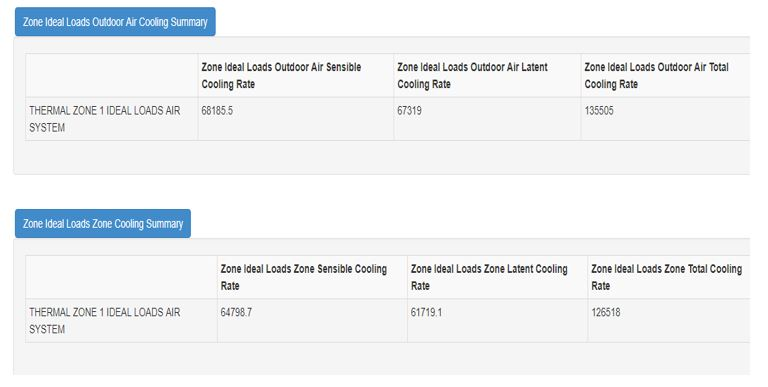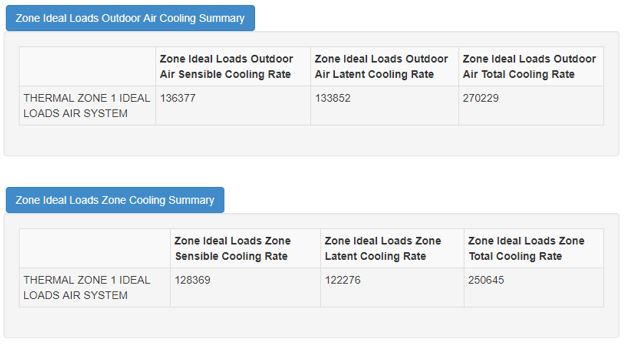Question-and-Answer Resource for the Building Energy Modeling Community
Get s tarted with the Help page
Hello everyone, I am using the Ideal Loads Air System ZoneHVAC:IdealLoadsAirSystem to calculate the zone cooling load for sizing a cooling coil. However, i have an issue on the output value of the "Zone Ideal Loads Zone Total Cooling Energy". "Zone Ideal Loads Zone Total Cooling Energy". I ran two simulation of the Ideal Loads Air System with different ventilation rate, and the ventilation rate of the second case is double of the first one. As expected, the output value of "Zone Ideal Loads Outdoor Air Total Cooling Energy" Energy" was doubled. However, what confused me was that the output value of "Zone Ideal Loads Zone Total Cooling Energy" "Zone Ideal Loads Zone Total Cooling Energy" had also nearly doubled. The two simulation had the same settings except the ventilation rate. My understanding is only the outdoor air load will change, while the Zone Ideal Loads Zone Total Cooling Energy Zone Ideal Loads Zone Total Cooling Energy should be unchanged. I have read the EnergyPlus document and know the several output variables related to the Ideal Load Air System follows the relation as below: Zone Ideal Loads Supply Air Total Cooling Energy + Zone Ideal Loads Heat Recovery Total Cooling Energy = Zone Ideal Loads Zone Total Cooling Energy+ Zone Ideal Loads Outdoor Air Total Cooling Energy I do not have heat recovery setting in my simulation. i cannot understand why the value of Zone Ideal Loads Zone Total Cooling Energy Zone Ideal Loads Zone Total Cooling Energy will change. The buidling is of single zone with 4 meters long and 4 meters wide with the the simulation performed under Guangzhou climate. The first simulation has OA flow rate of 10 m3/s and the load results are shown below:The second simulation has the OA flow rate of 20 m3/s and the load results are shown below:Hopefully you will give me some answers, Thank you guys.